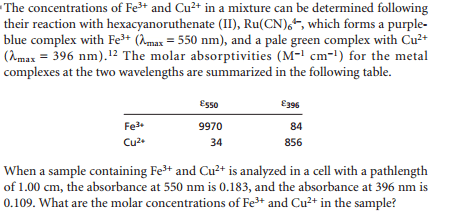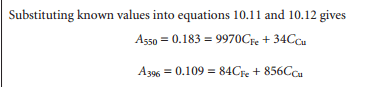# Chemistry [Spectrophotometry difficult example]

## Homework StatementA=epsilon*l*c

## The Attempt at a Solution

So I was wondering on how to set up the equations now, since I don't have much information? Which equations should i use to solve the problem?

Borek
Mentor
You have all the information needed. Assume the observed absorbance is the sum of individual absorbances.This is a big questionmark to me, why u should this?
0.183 = 9970*concentration of Fe + 34*concentration of cupper. Why is 0.183 equal to that?

Borek
Mentor
This is a direct application of the Lambert's Beer law which you wrote in your first post, and the fact that the observed absorbance is a sum of absorbances of copper and iron.

Assuming there is no copper, can you write formulas for the absorbances of the solution at both wavelengths? Can you do the same for iron? Can you add these values?

I still have really difficult! To understand why you add these values!

Borek
Mentor
I still have really difficult! To understand why you add these values!

Which part of the "absorbance is additive" statement is not clear to you?

If there is more than one substance present in the solution, each absorbs on its own, so the observed total absorbance is a sum of individual absorbances (at least for relatively diluted solutions).